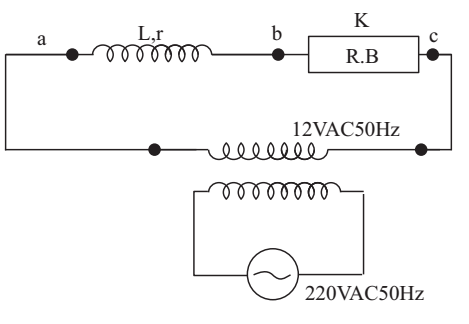# Inductance and Resistance of Coil Experiment

Experiment: Determine the inductance and resistance of a given coil (inductor) using a suitable series resistance and an AC voltmeter.

Depending upon its number of turns, area of cross-section of each turn, length of the coil and the permeability of the material of the core, every coil has certain self-inductance L.

In addition to its self-inductance, every coil has certain resistance r. For a given coil the value of r depends on the length of the wire, its area of cross-section (or thickness) and the resistivity of the material of the wire used for making the coil.

The rms voltages across the inductor and the series resistor R, as measured by an AC voltmeter will not be in phase and therefore cannot be added straight away to get the applied rms voltage.

Vapplied is the vector sum of VL and VR.

Material Required

A step-down transformer (its secondary windings to be used as an inductor), a resistance box, a voltmeter (range .0-15V), a step down transformer (220-12V AC 50Hz), connecting wires, a key, sand paper.

### How To Set Up

Clean the ends of the connecting wires with the sand paper and make tight connections as per the arrangement or circuit diagram.### How To Perform Experiment

1. After having assembled the circuit, check it once again with the circuit diagram.

2. Before inserting the plug in the key, take out R = 200 ohm plug from the resistance box.

3. Insert the plug in the key. Put the voltmeter first across the inductor and then across the resistance box. If needed, adjust R till the voltmeter reading is of the same order.

4. Read and record voltmeter readings VL and VR across L and R respectively. Put voltmeter across the series combination of L and R and read and record V.

5. Repeat step 4 above for 5 different values of R and record your observations in the observation table.

### Sources of Error

1. There may be error in the measurement of VR, VL, and Vappliedbecause of the finite least count of the voltmeter used.
2. During the course of measurement of VL and VR the applied voltage Vapplied may change due to fluctuations in the AC mains voltage.